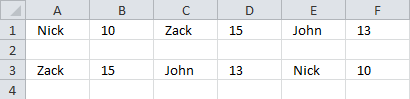Author: Oscar Cronquist Article last updated on July 25, 2017

I have a problem and i cant figure it out, even if i'm seraching for 2 days.
I have the following situation:
A1, B1, C1, D1, E1, F1
where
A1 = nick
b1 = 10
c1 = zack
d1 = 15
e1 - john
f1 = 13
what formula should i use to get them ordered counting the numbers but names still being asociated, like this:
a1 = zack
b1 = 15
c1 = john
d1 = 13
e1 = nick
f1 = 10Formula in cell A3:

=INDEX(\$A\$1:\$F\$1, MATCH(LARGE(\$A\$1:\$F\$1, ROUND(COLUMN(A1)*0.5, 0)), \$A\$1:\$F\$1, 0)-MOD(COLUMN(A1), 2))

### Explaining formula

Find n-th largest number in cell range and repeat every number

ROUND(COLUMN(A1)*0.5,0)

becomes ROUND(1*0.5,0)

becomes ROUND(0.5,0)

and returns 1. Copy the formula to the next cell gives ROUND(COLUMN(B1)*0.5,0) and returns 1 again.

As you copy the formula to the left this sequence is created 1,1,2,2,3,3,4,4,5,5 and so on.

What happens if we combine the above with the LARGE function?

LARGE(\$A\$1:\$F\$1,ROUND(COLUMN(A1)*0.5,0))

The LARGE function extracts the n-th largest number twice for every two cells. This allows us to return both the number and the corresponding name later on.

Find position of the n-th largest number in cell range

This formula is valid for cell A3, when you copy it to cell A4 the cell refs change. Check out blog post absolute and relative cell references and learn how to build smarter formulas.

MATCH(LARGE(\$A\$1:\$F\$1,ROUND(COLUMN(A1)*0.5,0)),\$A\$1:\$F\$1,0)

becomes

MATCH(15,\$A\$1:\$F\$1,0)

and returns 4. Read more about MATCH function.

Subtract one to get the corresponding name for every second cell.

MATCH(LARGE(\$A\$1:\$F\$1,ROUND(COLUMN(A1)*0.5,0)),\$A\$1:\$F\$1,0)-MOD(COLUMN(A1),2)

MOD(COLUMN(A1),2) returns 1,0,1,0,1, ... and so on as you copy the formula further to the right. This enables the formula to extract the name for every second cell.

More MOD examples: Learn how the MOD function worksQuotient, Mod and Int functions

Return number or name

=INDEX(\$A\$1:\$F\$1,MATCH(LARGE(\$A\$1:\$F\$1,ROUND(COLUMN(A1)*0.5,0)),\$A\$1:\$F\$1,0)-MOD(COLUMN(A1),2))

The INDEX function returns n-th largest number or corresponding name.

### Data verticallyThis formula returns data vertically:

=INDEX(\$A\$1:\$A\$6, MATCH(LARGE(\$A\$1:\$A\$6, ROUND(ROW(A1)*0.5, 0)), \$A\$1:\$A\$6, 0)-MOD(ROW(A1), 2))